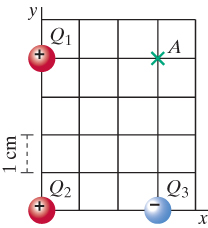# Problem: At a particular moment, three small charged balls, one negative and two positive,are located as shown in the figure. Q1 = 3 nC, Q2 = 5 nC, and Q3 = -4 nC.Remember that you must first convert all quantities to S.I. units. 1 nC = 1 nanocoulomb = 1e-9 C.(a1) What is the electric field at the location of Q1, due to Q2?E2 = ____ N/C(a2) What is the electric field at the location of Q1, due to Q3?E3 = ____ N/C(a3) What is the net electric field at the location of Q1, due to Q2 and Q3?Enet = ____ N/C(b) Use the electric field you calculated above to find the force on Q1 due to Q2 and Q3.F = ____ N(c1) What is the electric field at location A, due to Q1?EA,1 = ____ N/C(c2) What is the electric field at location A, due to Q2?EA,2 = ____ N/C(c3) What is the electric field at location A, due to Q3?EA,3 = ____ N/C(c4) What is the net electric field at location A?EA,net = ____ N/C(d) An alpha particle (He2+, containing two protons and two neutrons) is released from rest at location A. At the instant the particle is released, what is the electric force on the alpha particle, due to Q1, Q2 and Q3?F = ____ N

###### FREE Expert Solution

Electric field:

$\overline{){\mathbf{E}}{\mathbf{=}}\frac{\mathbf{k}\mathbf{q}}{{\mathbf{r}}^{\mathbf{2}}}}$, where k is Coulomb's constant, q is the charge, and r is the distance from the point to the charge.

(a1)

We get the electric field as:

$\begin{array}{rcl}{\mathbf{E}}_{\mathbf{2}}& \mathbf{=}& \frac{\mathbf{k}{\mathbf{Q}}_{\mathbf{2}}}{{{\mathbf{r}}_{\mathbf{12}}}^{\mathbf{2}}}\\ & \mathbf{=}& \frac{\mathbf{\left(}\mathbf{9}\mathbf{×}{\mathbf{10}}^{\mathbf{9}}\mathbf{\right)}\mathbf{\left(}\mathbf{5}\mathbf{×}{\mathbf{10}}^{\mathbf{-}\mathbf{9}}\mathbf{\right)}}{{\mathbf{\left(}\mathbf{4}\mathbf{×}{\mathbf{10}}^{\mathbf{-}\mathbf{2}}\mathbf{\right)}}^{\mathbf{2}}}\end{array}$, this is because there are four boxes between Q1 and Q2 and each box is 1 cm.

98% (466 ratings)###### Problem Details

At a particular moment, three small charged balls, one negative and two positive,are located as shown in the figure. Q1 = 3 nC, Q2 = 5 nC, and Q3 = -4 nC.

Remember that you must first convert all quantities to S.I. units. 1 nC = 1 nanocoulomb = 1e-9 C.(a1) What is the electric field at the location of Q1, due to Q2?

E2 = ____ N/C

(a2) What is the electric field at the location of Q1, due to Q3?

E3 = ____ N/C

(a3) What is the net electric field at the location of Q1, due to Q2 and Q3?

Enet = ____ N/C

(b) Use the electric field you calculated above to find the force on Q1 due to Q2 and Q3.

F = ____ N

(c1) What is the electric field at location A, due to Q1?

EA,1 = ____ N/C

(c2) What is the electric field at location A, due to Q2?

EA,2 = ____ N/C

(c3) What is the electric field at location A, due to Q3?

EA,3 = ____ N/C

(c4) What is the net electric field at location A?

EA,net = ____ N/C

(d) An alpha particle (He2+, containing two protons and two neutrons) is released from rest at location A. At the instant the particle is released, what is the electric force on the alpha particle, due to Q1, Q2 and Q3?

F = ____ N